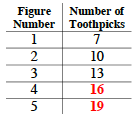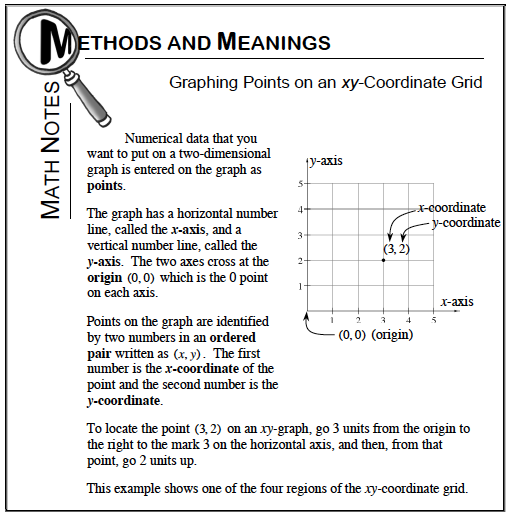Home > MC1 > Chapter 8 > Lesson 8.2.3 > Problem8-48

8-48.

Julian was studying a pattern made with toothpicks, and he started the table shown below.

Figure Number

Number of Toothpicks

1

7

2

10

3

13

4

5

1. Copy and complete the table.

2. Draw axes and plot Julian's points.

3. How can you describe what all of these points have in common?

Do you notice the pattern Julian made? In figures 1, 2, and 3, the number of toothpicks increase by 3. Can you complete the table?

The completed table should look like the one below.It may help to label your x-axis (horizontal) ''Figure Number'' and your y-axis (vertical) ''Number of Toothpicks''.

For a review of your coordinate point knowledge, refer to the Math Notes box below.Try taking a look at your graph. Do your points form any kind of figure or line?